How to build polyhedra with cardboard (plane nets)

Cardoboard is a wonderful material to build polyhedra. One approach is to draw a plane net of a polyhedron.

Some tricks when we use cardboard:Cube and tetrahedron

Plane nets of a tetrahedron and a cube are very easy to draw.This construction was drawn by Kepler. We can use it to calculate the volume of a tetrahedron:The volume of a tetrahedron is one third of the prism that contains it.

How to build a tetrahedron like TetraPack.Hexagonal section of a cube

You can cut a cube by half and get a regular hexagon:We can cut in half a cube by a plane and get a section that is a regular hexagon. Using eight of this pieces we can made a truncated octahedron.
Template of a half cube to dowload, print, cut and fold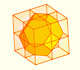Using eight half cubes we can make a truncated octahedron. The cube tesselate the space an so do the truncated octahedron. We can calculate the volume of a truncated octahedron.These polyhedra pack together to fill space, forming a 3 dimensional space tessellation or tilling.
Plane net of a dodecahedron

Very nice construction.

Durer was the first to publish a plane net of a dodecahedron: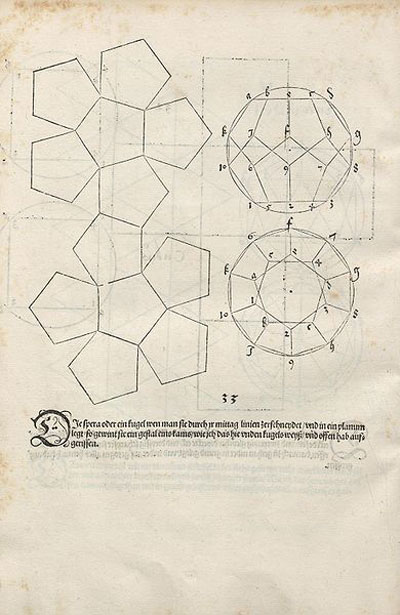Kepler was very interested in the dodecahedron: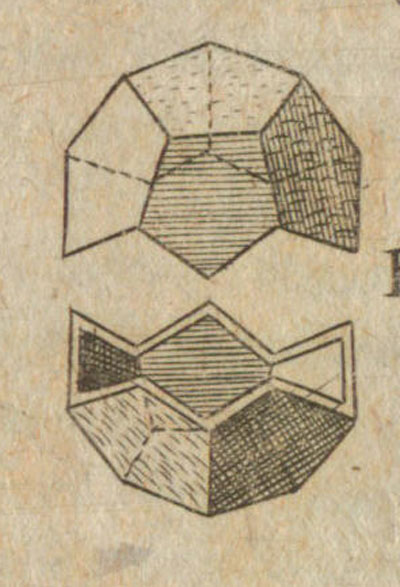Puzzle: A tetrahedron with two pieces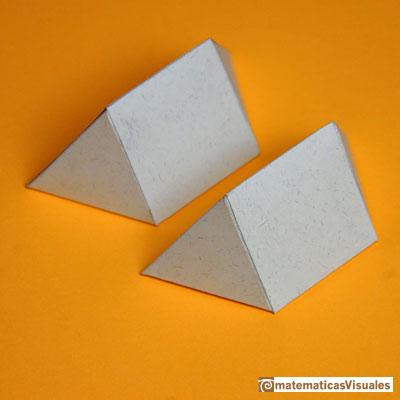With these two pieces you can build a tetrahedron.

These pieces are made of two tetrahedron and half octahedron. Can you see them?

REFERENCES

Magnus Wenninger - 'Polyhedron Models', Cambridge University Press.
Hugo Steinhaus - Mathematical Snapshots - Oxford University Press - Third Edition (p. 197)
Peter R. Cromwell - 'Polyhedra', Cambridge University Press, 1999.
H.Martin Cundy and A.P. Rollet, 'Mathematical Models', Oxford University Press, Second Edition, 1961 (p. 87).
W.W. Rouse Ball and H.S.M. Coxeter - 'Matematical Recreations & Essays', The MacMillan Company, 1947.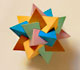Using cardboard you can build beautiful polyhedra cutting polygons and glue them toghether. This is a very simple and effective technique. You can download several templates. Then print, cut and glue: very easy!Simple technique to build polyhedra gluing discs made of cardboard or paper.Italian designer Bruno Munari conceived 'Acona Biconbi' as a work of sculpture. It is also a beautiful game to play with colors and shapes.Material for a session about polyhedra (Zaragoza, 9th May 2014). Simple techniques to build polyhedra like the tetrahedron, octahedron, the cuboctahedron and the rhombic dodecahedron. We can build a box that is a rhombic dodecahedron.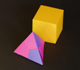Material for a session about polyhedra (Zaragoza, 7th November 2014). We study the octahedron and the tetrahedron and their volumes. The truncated octahedron helps us to this task. We build a cubic box with cardboard and an origami tetrahedron.Material for a session about polyhedra (Zaragoza, 23rd Octuber 2015) . Building a cube with cardboard and an origami octahedron.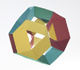Material for a session about polyhedra (Zaragoza, 21st October 2016). Instructions to build several geometric bodies.Some properties of this platonic solid and how it is related to the golden ratio. Constructing dodecahedra using different techniques.The first drawing of a plane net of a regular dodecahedron was published by Dürer in his book 'Underweysung der Messung' ('Four Books of Measurement'), published in 1525 .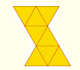The first drawing of a plane net of a regular octahedron was published by Dürer in his book 'Underweysung der Messung' ('Four Books of Measurement'), published in 1525 .The first drawing of a plane net of a regular tetrahedron was published by Dürer in his book 'Underweysung der Messung' ('Four Books of Measurement'), published in 1525 .A cuboctahedron is an Archimedean solid. It can be seen as made by cutting off the corners of a cube.The truncated tetrahedron is an Archimedean solid made by 4 triangles and 4 hexagons.We study different cylinders and we can see how they develop into a plane. Then we explain how to calculate the lateral surface area.Plane developments of cones and conical frustum. How to calculate the lateral surface area.The first drawing of a plane net of a regular dodecahedron was published by Dürer in his book 'Underweysung der Messung' ('Four Books of Measurement'), published in 1525 .